# Build Textual Similarity Analysis Web App

##### A journey from word embeddings to universal sentence encoder to web app demoHave you wondered how search engines understand your queries and retrieve relevant results? How chatbots extract your intent from your questions and provide the most appropriate response?

In this story, I will detail each part needed to build a textual similarity analysis web-app:

• word embeddings
• sentence embeddings
• cosine similarity
• build a textual similarity analysis web-app
• analysis of results

Try the textual similarity analysis web-app, and let me know how it works for you in the comments below!

# Word embeddings

Word embeddings enable knowledge representation where a vector represents a word. This improves the ability for neural networks to learn from a textual dataset.

Before word embeddings were de facto standard for natural language processing, a common approach to deal with words was to use a one-hot vectorisation. Each word represents a column in the vector space, and each sentence is a vector of ones and zeros. Ones denote the presence of the word in the sentence.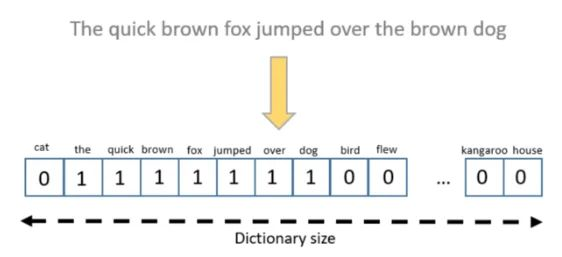As a result, this leads to a huge and sparse representation, because there are much more zeros than ones. When there are many words in the vocabulary, this creates a large word vector. This might become a problem for machine learning algorithms.

One-hot vectorisation also fails to capture the meaning of words. For example, “drink” and “beverage”, even though these are two different words, they have a similar definition.

With word embeddings, semantically similar words have similar vectors representation. As a result, “I would like to order a drink” or “a beverage”, an ordering system can interpret that request the same way.

## In the past

Back in 2003, Yoshua Bengio et al. introduced a language model concept. The focus of the paper is to learn representations for words, which allow the model to predict the next word.

This paper is crucial and led to the development to discover word embeddings. Yoshua received the Turing Award alongside with Geoffrey Hinton, and Yann LeCun.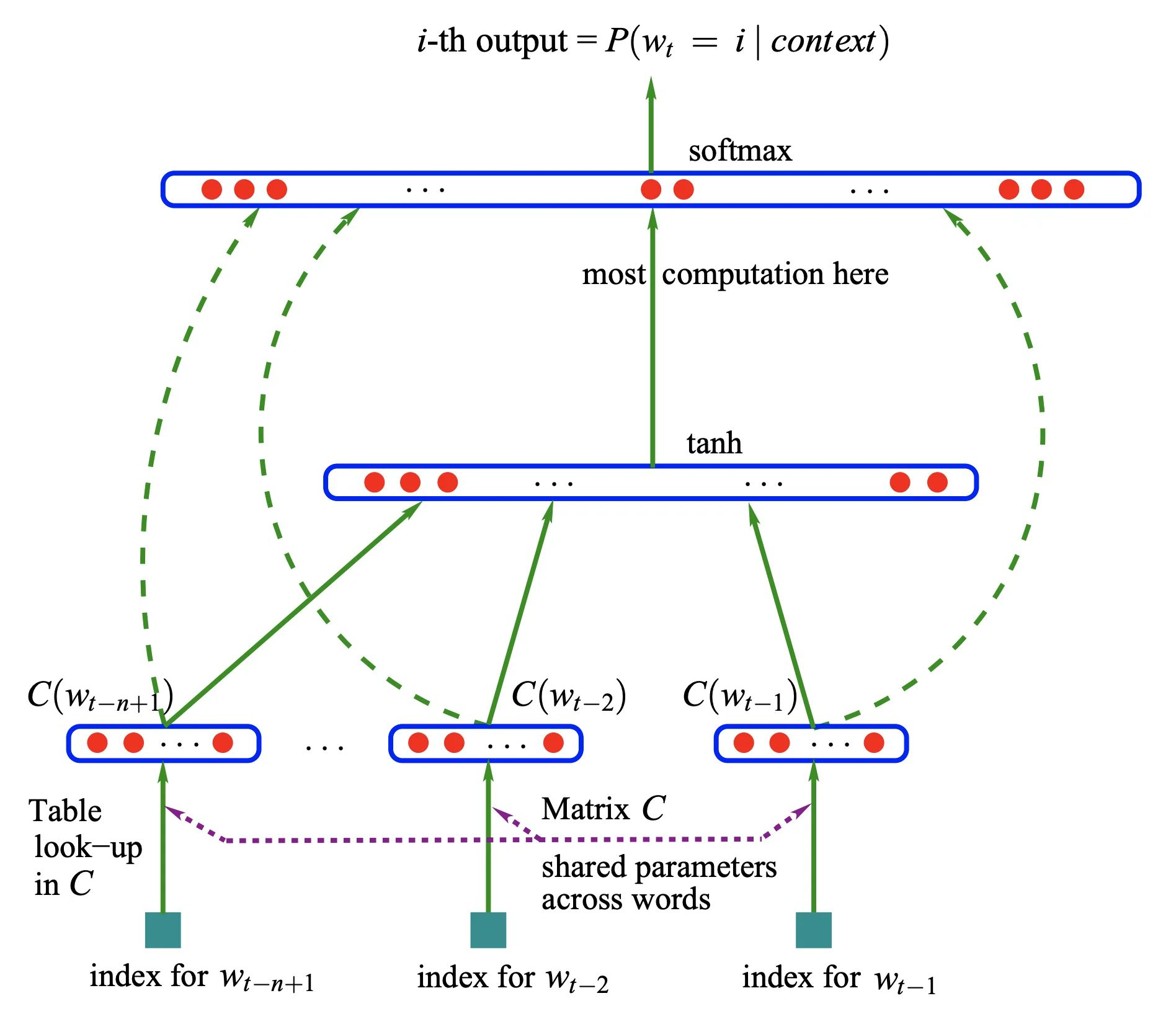In 2008, Ronan and Jason worked on a neural network that could learn to identify similar words. Their discovery has opened up many possibilities for natural language processing. The table below shows a list of words and the respective ten most similar words.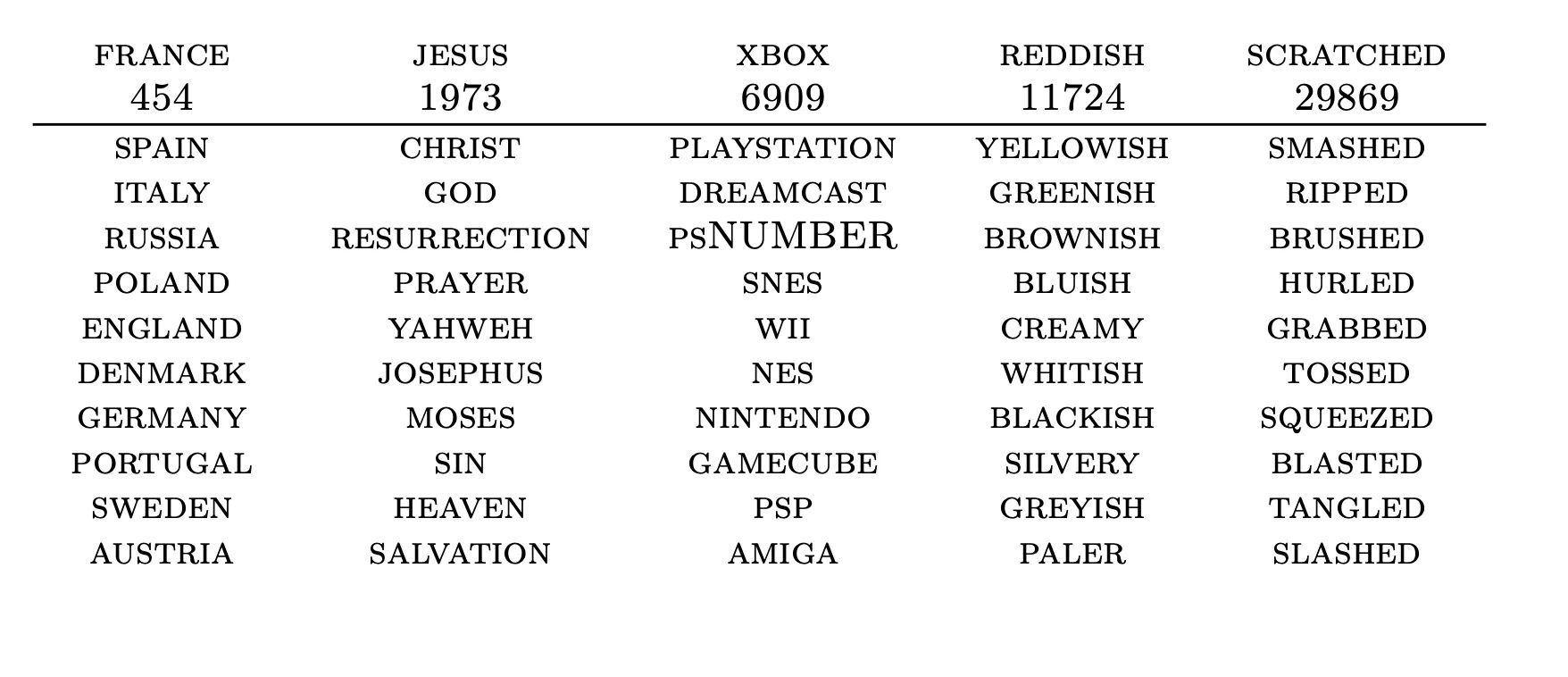In 2013, Tomas Mikolov et al. introduced learning high-quality word vectors from datasets with billions of words. They named it Word2Vec, and it contains millions of words in the vocabulary.

Word2Vec has become popular since then. Nowadays, the word embeddings layer is in all popular deep learning framework.

## Examples

On Google’s pretrained Word2Vec model, they trained on roughly 100 billion words from Google News dataset. The word “cat” shares the closest meanings to “cats”, “dog”, “mouse”, “pet”.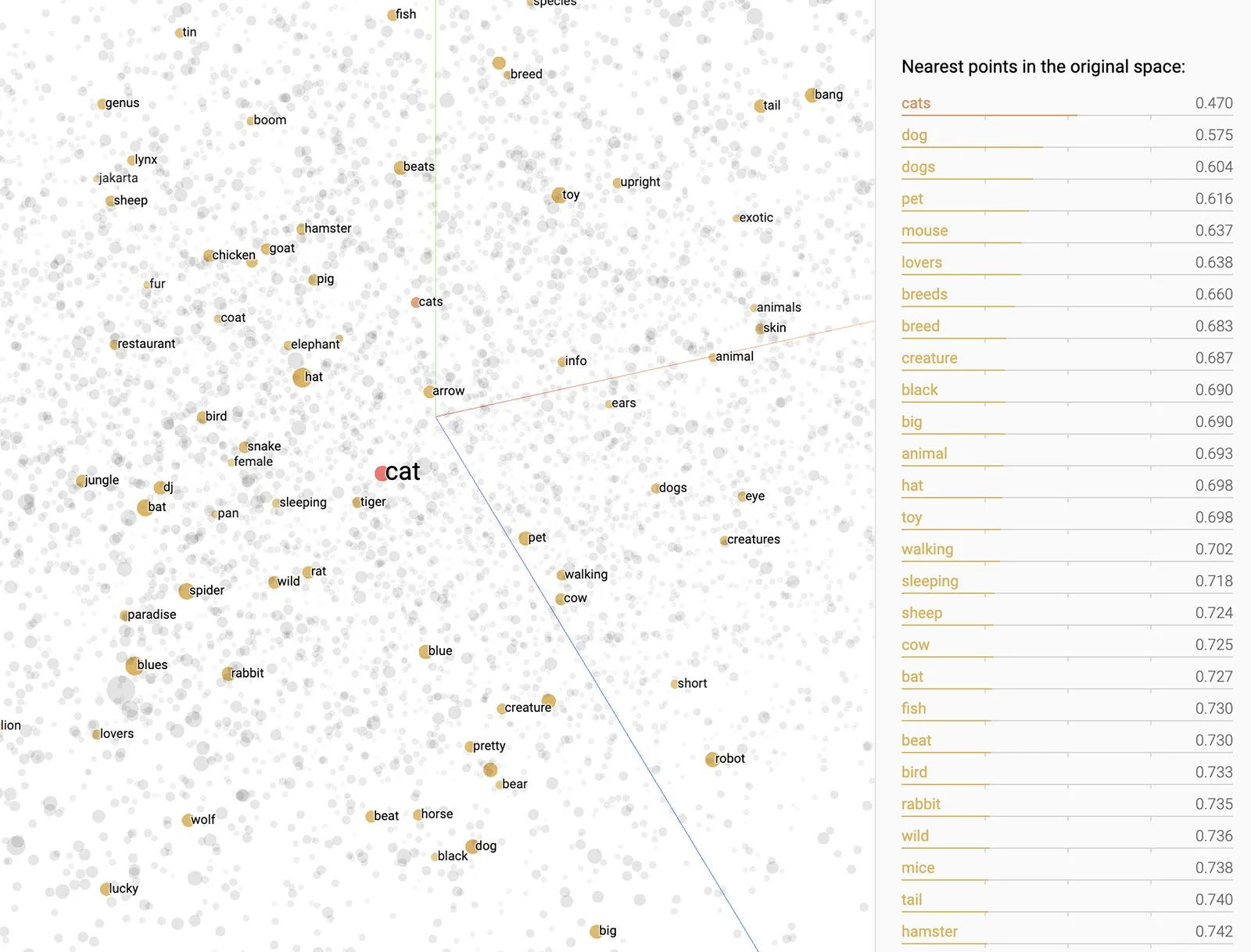Word embedding also manages to recognise relationships between words. A classic example is the gender-role relationships between words. For example, “man” is to “woman” is like “king” is to “queen”.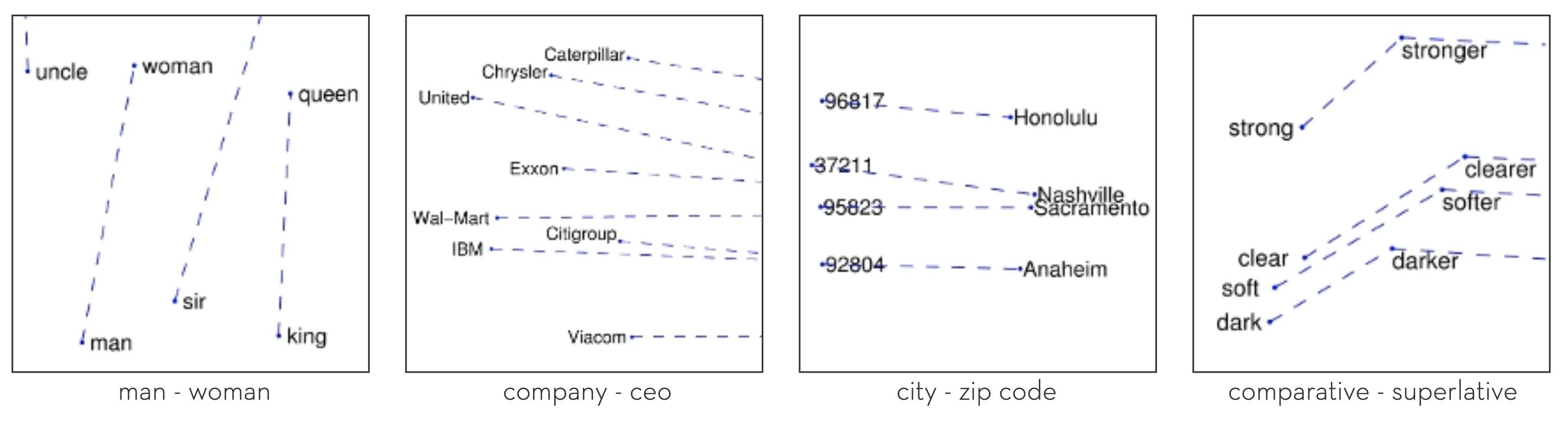## Dig deeper

Galina Olejnik did an excellent job describing the motivation of word embeddings. From one-hot encoding and TF-IDF to GloVe and Poincaré.

Word embeddings: exploration, explanation, and exploitation (with code in Python)

Here’s a 29-minute comprehensive article about various language models by Dipanjan (DJ) Sarkar. He covers Word2Vec, GloVe and FastText; do check this out, if you are planning to work on word embeddings.

A hands-on intuitive approach to Deep Learning Methods for Text Data — Word2Vec, GloVe and FastText

## Do it yourself

TensorFlow has provided a tutorial on word embeddings and codes in this Colab notebook. You can get your hands dirty with the codes and use it to train your word embeddings on your dataset. This can definitely help you get started.

For who enjoys animation, there is a cool embeddings visualisation on Embedding Projector. Every dot represents a word, and you can visualise semantically similar words in a 3D space.

We have word vectors to represent meanings for words; how about sentences?

# Universal Sentence Encoder

Like word embeddings, universal sentence encoder is a versatile sentence embedding model that converts text into semantically-meaningful fixed-length vector representations.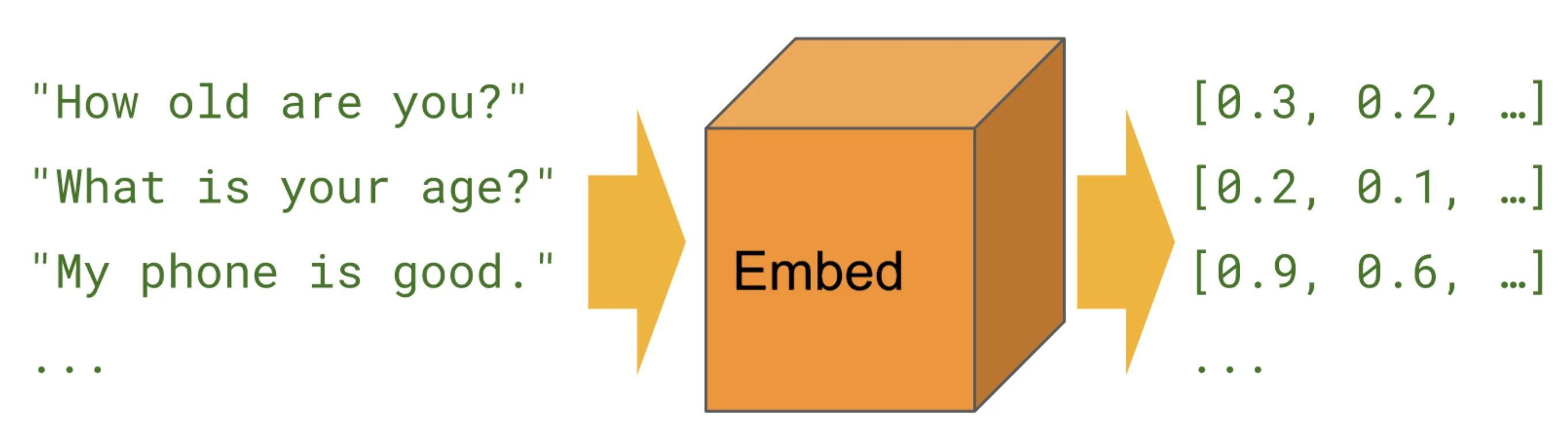These vectors produced by the universal sentence encoder capture rich semantic information. We can use it for various natural language processing tasks, to train classifiers such as classification and textual similarity analysis.

There are two universal sentence encoder models by Google. One of them is based on a Transformer architecture and the other one is based on Deep Averaging Network.

Transformer, the sentence embedding creates context-aware representations for every word to produce sentence embeddings. It is designed for higher accuracy, but the encoding requires more memory and computational time. This is useful for sentiment classification where words like ‘not’ can change the meaning and able to handle double negation like “not bad”.

Deep Averaging Network, the embedding of words are first averaged together and then passed through a feedforward deep neural network to produce sentence embeddings. Unfortunately, by averaging the vectors, we lose the context of the sentence and sequence of words in the sentence in the process. It is designed for speed and efficiency, and some accuracy is sacrificed (especially on sarcasm and double negation). A great model for topic classification, classifying long articles into categories.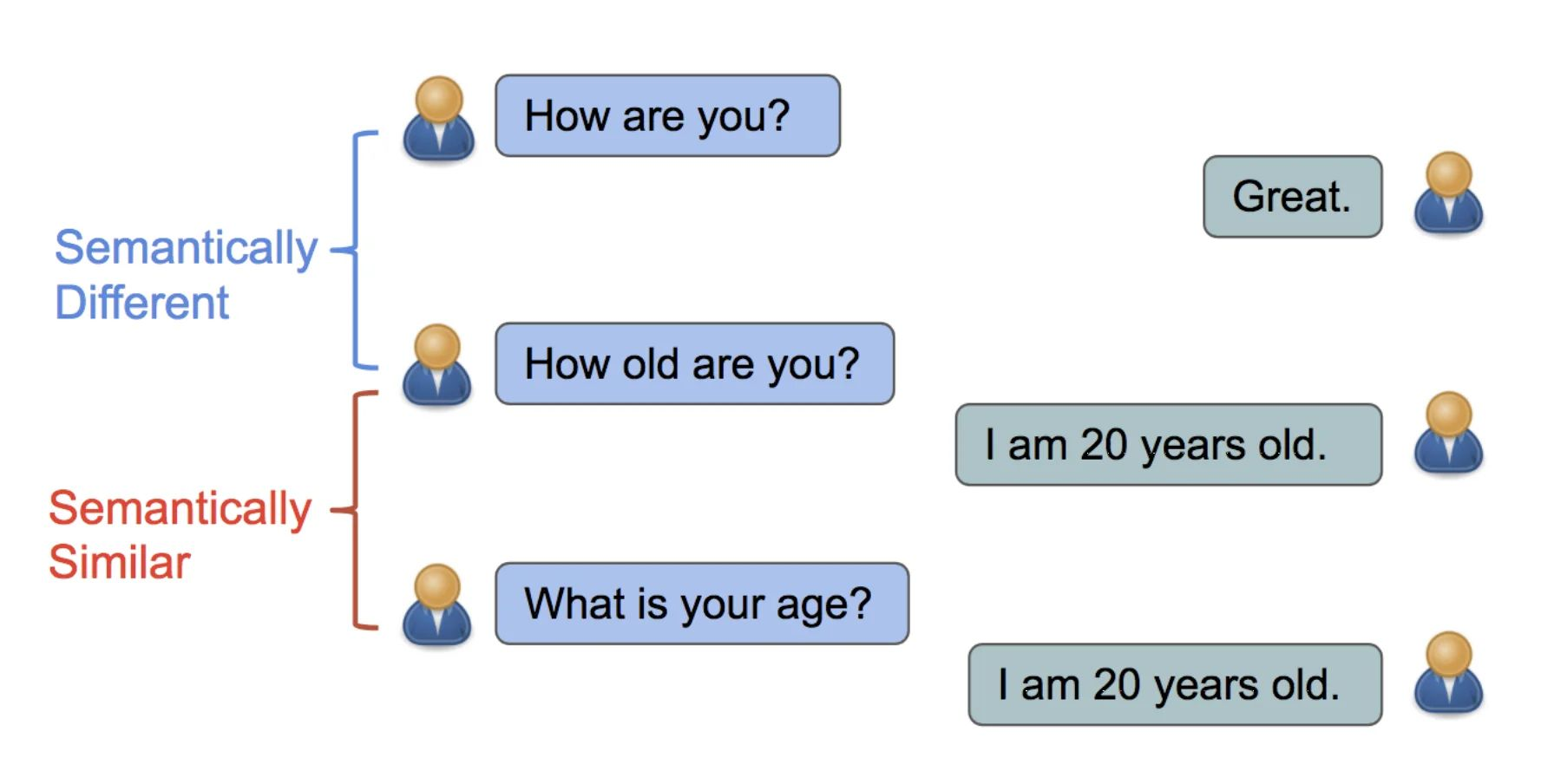Yinfei Yang et al. introduce a way to learn sentence representations using conversational data.

For example, “How old are you?” and “What is your age?”, both questions are semantically similar, a chatbot can reply the same answer “I am 20 years old”.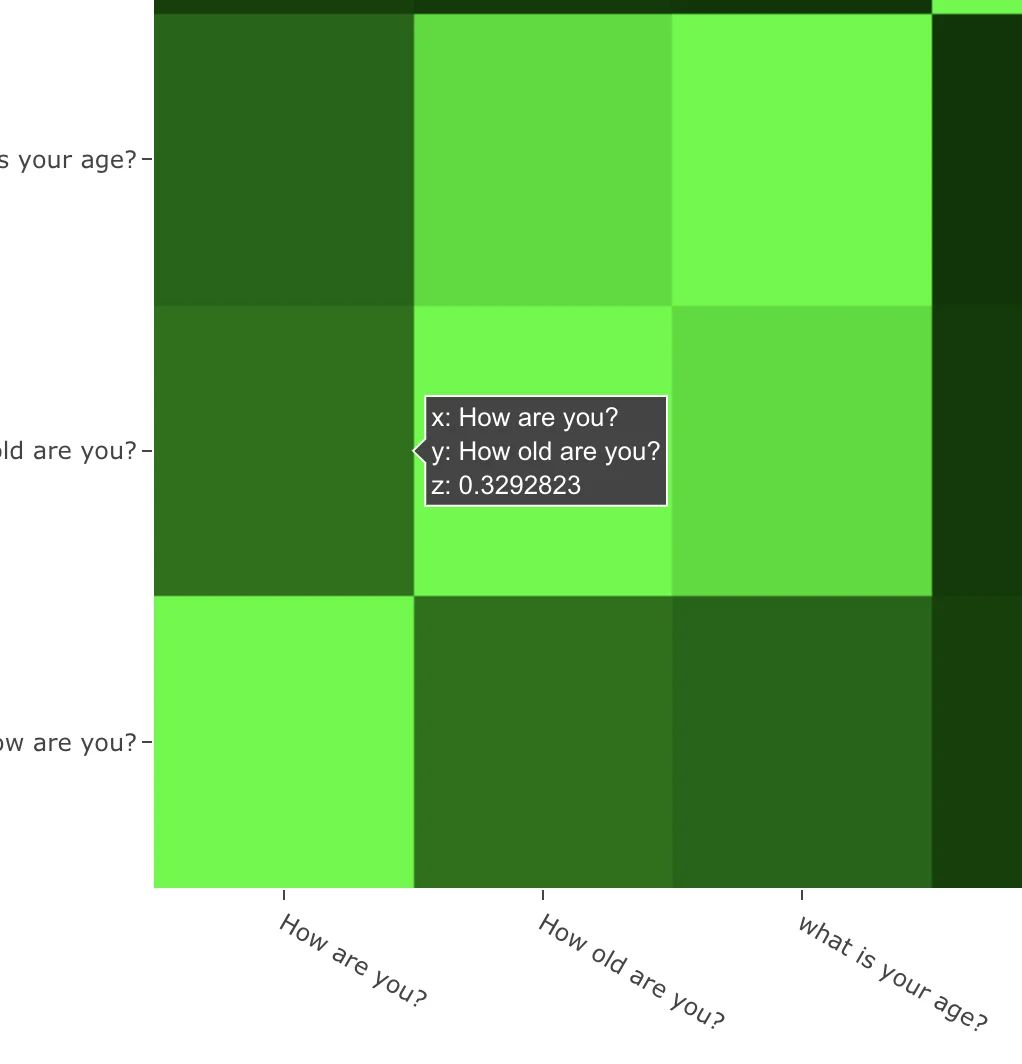In contrast, while “How are you?” and “How old are you?” contain identical words, both sentences have different meanings. A chatbot has to understand the question and provide the appropriate response.

This is a heatmap showing the similarity between three sentences “How old are you?”, “What is your age?” and “How are you?”.

How are you?” and “How old are you?” have low similarity score even though having identical words.

Logeswaran et al. introduced a framework to learn sentence representations from unlabelled data. In this paper, the decoder (orange box) used in prior methods is replaced with a classifier that chooses the target sentence from a set of candidate sentences (green boxes); it improves the performance of question and answer system.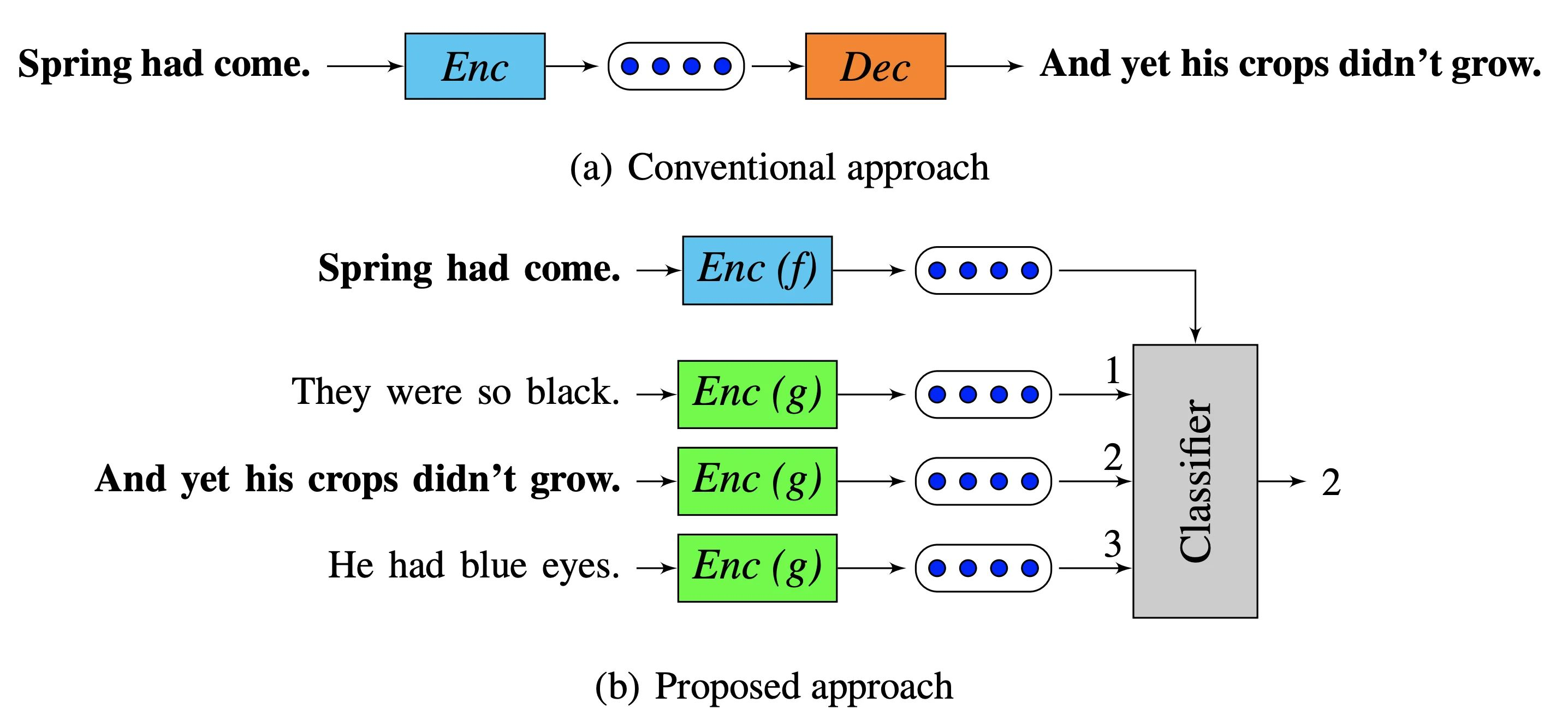## Dig deeper

Dipanjan (DJ) Sarkar explained the development of each embedding models. If you are keen to build a text classifier, his article detailed each step to perform sentiment analysis on movie reviews dataset.

Deep Transfer Learning for Natural Language Processing — Text Classification with Universal

If you are curious to explore other language models, Pratik Bhavsar compared the performance of various language models such as BERT, ELMo, USE, Siamese and InferSent. Learning to choose the correct one will improve the outcome of your results.

Variety Of Encoders In NLP

## Do it yourself

TensorFlow has provided a tutorial, a pretrained model and a notebook on universal sentence encoder. Definitely check this out if you are thinking about building your own text classifier.

With semantically-meaningful vectors for each sentence, how can we measure the similarity between sentences?

# Cosine similarityCosine similarity is a measure of similarity by calculating the cosine angle between two vectors. If two vectors are similar, the angle between them is small, and the cosine similarity value is closer to 1.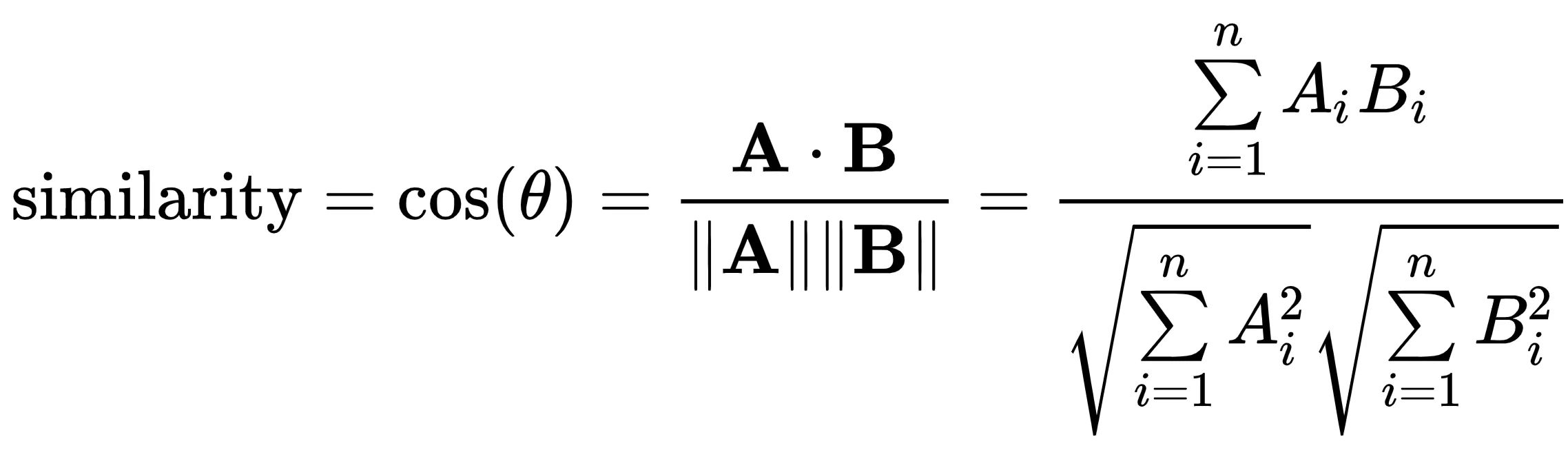Given two vectors A and B, the cosine similarity, cos(θ), is represented using a dot product and magnitude [from Wikipedia]

Here we input sentences into the universal sentence encoder, and it returns us sentence embeddings vectors.

With the vectors, we can take the cosine similarities between vectors. For every sentence pair, A and B, we can calculate the cosine similarity of A and B vectors.We can determine a minimum threshold to group sentence together. As similarity score falls between 0 to 1, perhaps we can choose 0.5, at the halfway mark. That means any sentence that is greater than 0.5 similarities will be cluster together.

## Dig deeper

Euge Inzaugarat introduced six methods to measure the similarity between vectors. Each method is suitable for a particular context, so knowing them it’s like knowing your data science toolbox well.

How to measure distances in machine learning

# Building blocks of a textual similarity analysis web-appIn this project, I will be using these libraries:

• TensorFlow.js
• Universal sentence encoder
• Angular

## TensorFlow.js

TensorFlow.js is a framework built by Google which enables machine learning in JavaScript. We can develop machine learning models and deploy them in the web browser and Node.js.

As I enjoy developing web applications, I was so happy when TensorFlow.js released in 2018.

It is easy to get started, and we can install TensorFlow.js with npm.

``````\$ npm install @tensorflow/tfjs
``````

An example of a simple linear regression model would look like this.

``````import * as tf from '@tensorflow/tfjs';

const model = tf.sequential();

model.compile({loss: 'meanSquaredError', optimizer: 'sgd'});

const xs = tf.tensor2d([1, 2, 3, 4], [4, 1]);
const ys = tf.tensor2d([1, 3, 5, 7], [4, 1]);

model.fit(xs, ys, {epochs: 10}).then(() => {
model.predict(tf.tensor2d(, [1, 1])).print();
});
``````

## Universal sentence encoder

I will be using is the universal sentence encoder package from TensorFlow.js. We can install universal sentence encoder using npm.

``````\$ npm install @tensorflow-models/universal-sentence-encoder
``````

This is an example to show how we can extract embeddings from each sentence using universal sentence encoder.

``````import * as use from '@tensorflow-models/universal-sentence-encoder';

const sentences = [
'Hello.',
'How are you?'
];
model.embed(sentences).then(embeddings => {
embeddings.print(true /* verbose */);
});
});
``````

## Angular

Angular is a web application framework built by Google for creating dynamic single-page apps.

For this project, I am using Angular 8.0. I enjoy building on Angular for its model–view–controller design pattern. I have used Angular since its first version and for most of my web development. But since they roll out major releases every half a year, feeling that my work will become obsolete (maybe? I don’t know). React is a popular UI framework, so perhaps I might switch to React one day. Who knows?

## Cosine similarity

Create a function to calculate the similarity of two vectors using the cosine similarity formula.

``````similarity(a, b) {
var magnitudeA = Math.sqrt(this.dot(a, a));
var magnitudeB = Math.sqrt(this.dot(b, b));
if (magnitudeA && magnitudeB)
return this.dot(a, b) / (magnitudeA * magnitudeB);
else return false
}
``````

Another function to calculate the similarity scores for every sentence pair as follows.

``````cosine_similarity_matrix(matrix){
let cosine_similarity_matrix = [];
for(let i=0;i<matrix.length;i++){
let row = [];
for(let j=0;j<i;j++){
row.push(cosine_similarity_matrix[j][i]);
}
row.push(1);
for(let j=(i+1);j<matrix.length;j++){
row.push(this.similarity(matrix[i],matrix[j]));
}
cosine_similarity_matrix.push(row);
}
return cosine_similarity_matrix;
}
``````

## Combine everything together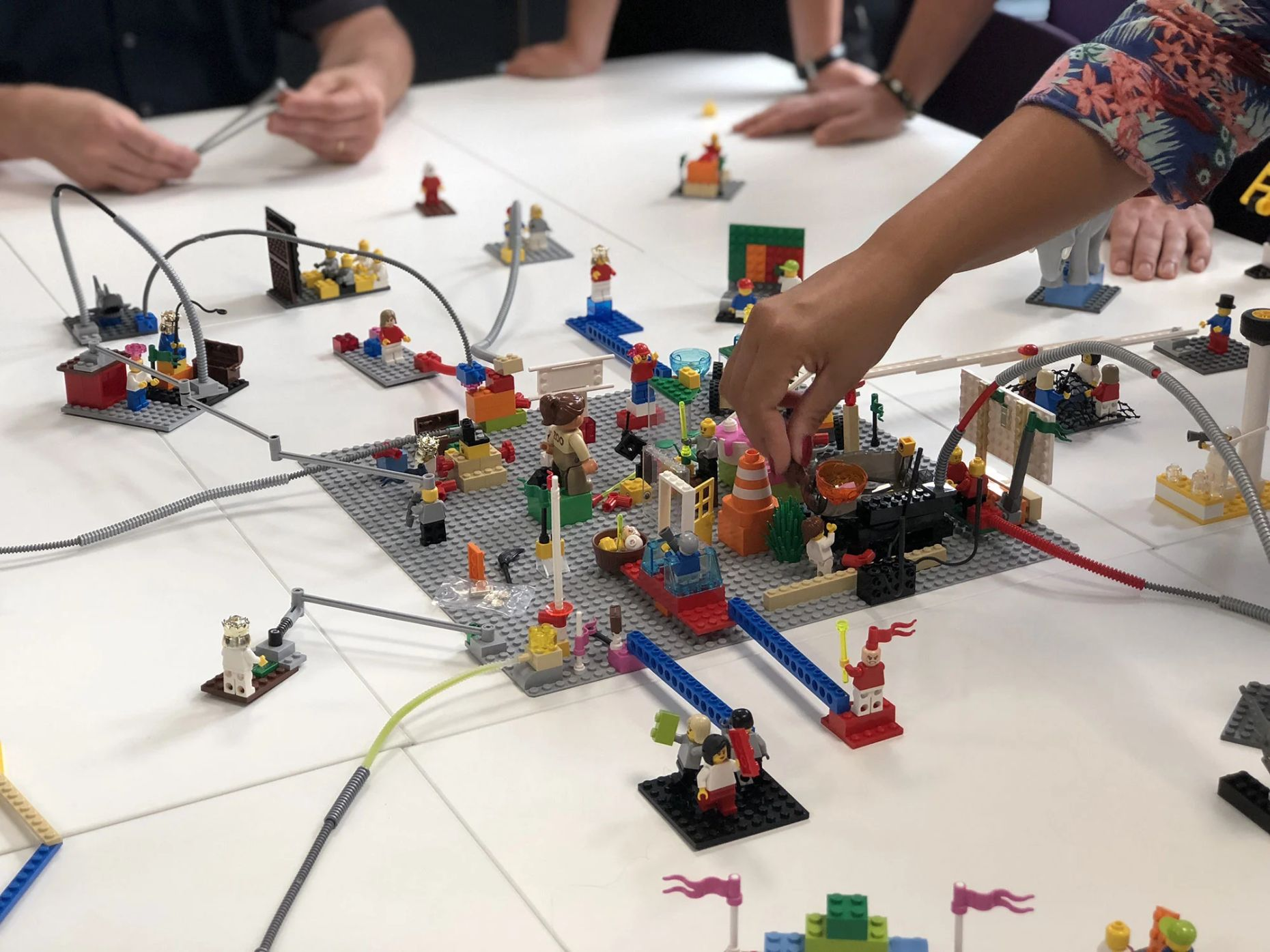I have introduced all the major components needed for this project. Now we just have to stack them up like Legos, package it and deploy to Github.

Voilà! We get a web application for a live demo.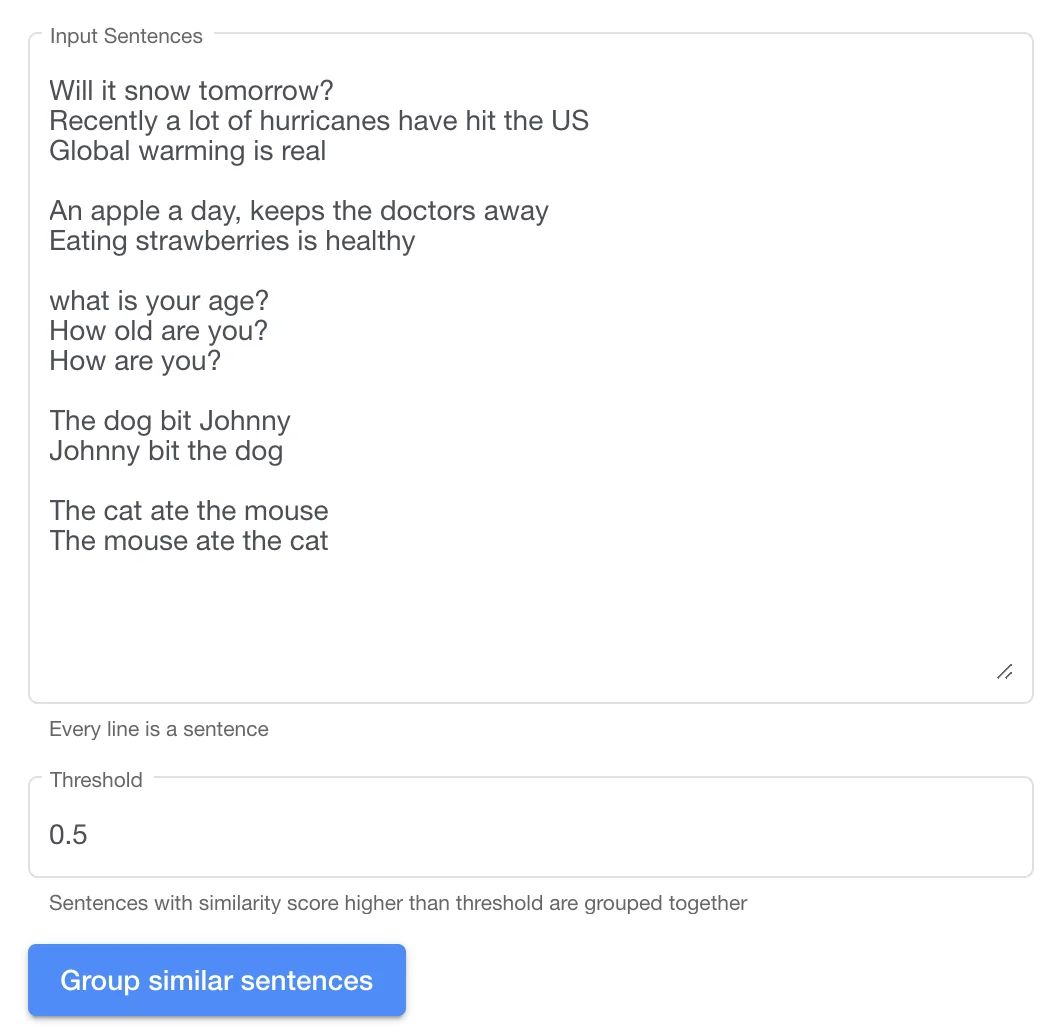We have a list of sentences, and these will be input into the universal sentence encoder. It will output will the embeddings of each sentence. Then we calculate the similarity between each sentence.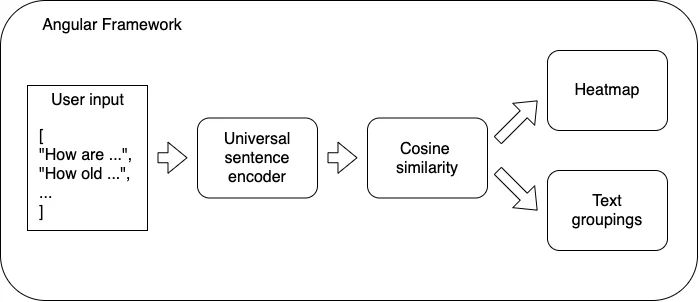## Results

These are the sentences we will be testing our universal sentence encoder. The objective is to group sentences with similar meaning together. I have picked a few difficult cases, so let us see how it performs.

Will it snow tomorrow?
Recently a lot of hurricanes have hit the US
Global warming is real

An apple a day, keeps the doctors away
Eating strawberries is healthy

How old are you?
How are you?

The dog bit Johnny
Johnny bit the dog

The cat ate the mouse
The mouse ate the cat

This heatmap shows how similar each sentence are to other sentences. The brighter the green represents similarity closer to 1, which means the sentences are more identical to each other.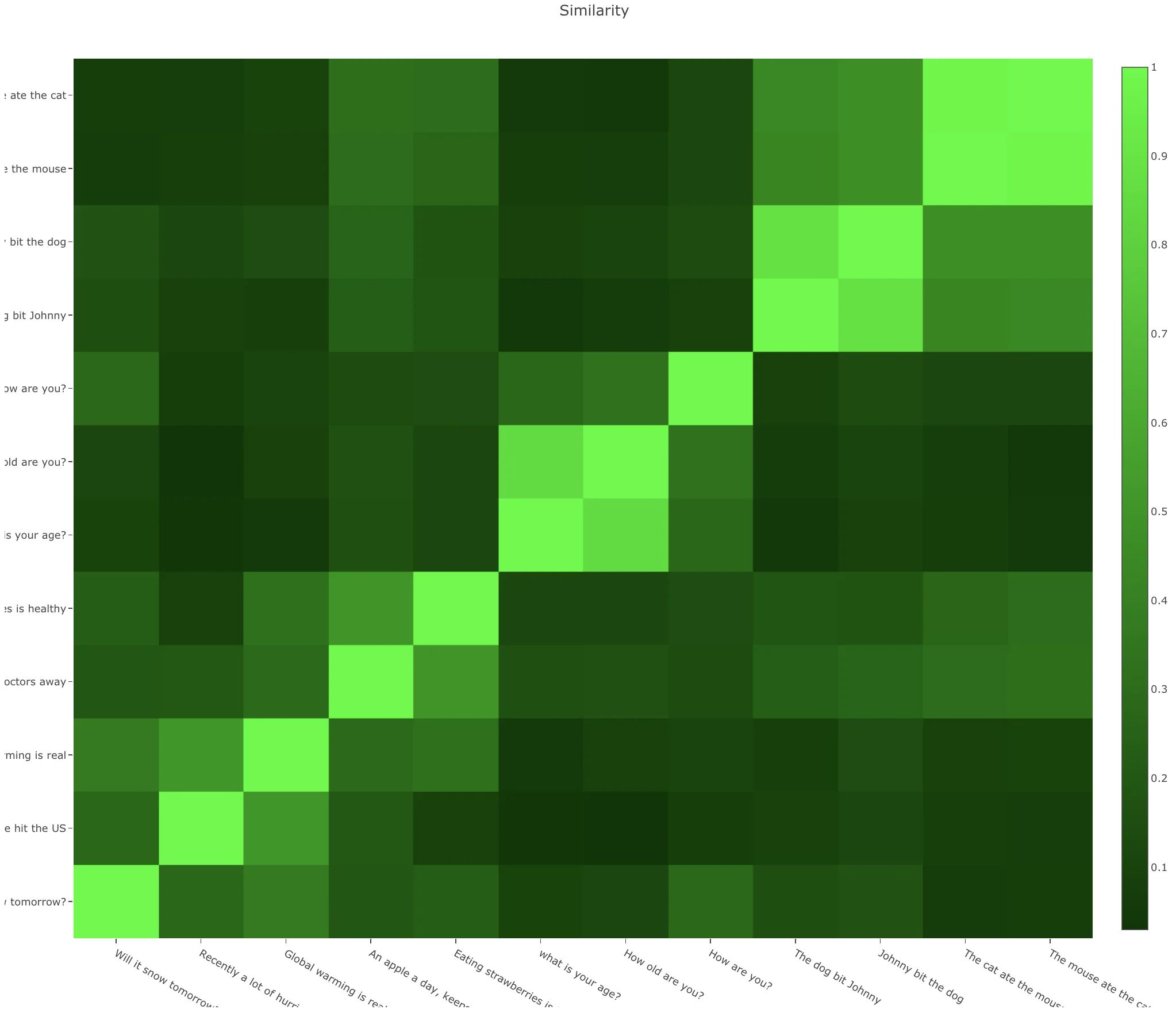We can adjust the value to determine a minimum similarity threshold to group sentences together. These are the sentences grouped together with greater than 0.5 similarity value.

Group 1
Recently a lot of hurricanes have hit the US
Global warming is real

Group 2
An apple a day, keeps the doctors away
Eating strawberries is healthy

Group 3
How old are you?

Group 4
The dog bit Johnny
Johnny bit the dog

Group 5
The cat ate the mouse
The mouse ate the cat

Our web application did an excellent job recognising “Group 1” being weather-related issues. Even though both sentences do not have any overlapping words.

It managed to identify that “hurricanes” and “global warming” are weather-related, but somehow didn’t manage to group the “snow” into this category.

Unfortunately, “Johnny bit the dog” and “The dog bit Johnny” has an 87% similarity. Poor Johnny, I don’t know which is better.

Likewise for “The cat ate the mouse” and “The mouse ate the cat”, I would be expecting that the two vectors to have an opposing similarity.

Once again, do try the textual similarity analysis web-app, and let me know how it works for you in the comments below!

Check out the codes for the web application if you would like to build something similar.

# Other machine learning web applications I’ve built

Time Series Forecasting with TensorFlow.js

Reinforcement Learning Value Function